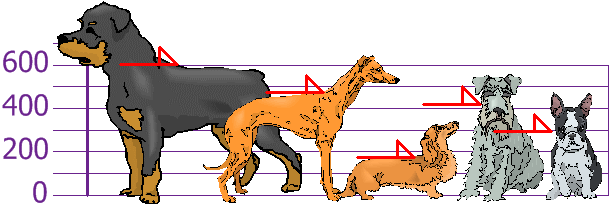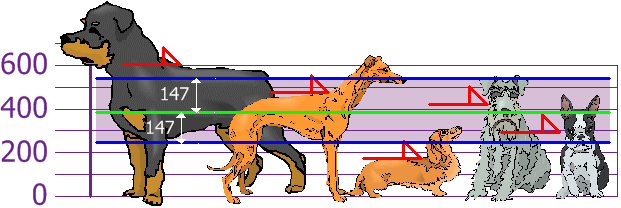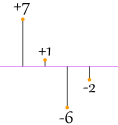# Standard Deviation and Variance

Deviation just means how far from the normal

## Standard Deviation

The Standard Deviation is a measure of how spread out numbers are.

Its symbol is σ (the greek letter sigma)

The formula is easy: it is the square root of the Variance. So now you ask, "What is the Variance?"

## Variance

The Variance is defined as:

The average of the squared differences from the Mean.

To calculate the variance follow these steps:

• Work out the Mean (the simple average of the numbers)
• Then for each number: subtract the Mean and square the result (the squared difference).
• Then work out the average of those squared differences. (Why Square?)

## Example

You and your friends have just measured the heights of your dogs (in millimeters):The heights (at the shoulders) are: 600mm, 470mm, 170mm, 430mm and 300mm.

Find out the Mean, the Variance, and the Standard Deviation.

Your first step is to find the Mean:

 Mean = 600 + 470 + 170 + 430 + 3005 = 19705 = 394

so the mean (average) height is 394 mm. Let's plot this on the chart:Now we calculate each dog's difference from the Mean:To calculate the Variance, take each difference, square it, and then average the result:

 Variance σ2 = 2062 + 762 + (−224)2 + 362 + (−94)25 = 42436 + 5776 + 50176 + 1296 + 88365 = 1085205 = 21704

So the Variance is 21,704

And the Standard Deviation is just the square root of Variance, so:

 Standard Deviation σ = √21704 = 147.32... = 147 (to the nearest mm)

And the good thing about the Standard Deviation is that it is useful. Now we can show which heights are within one Standard Deviation (147mm) of the Mean:So, using the Standard Deviation we have a "standard" way of knowing what is normal, and what is extra large or extra small.

Rottweilers are tall dogs. And Dachshunds are a bit short, right?

## UsingWe can expect about 68% of values to be within plus-or-minus 1 standard deviation.

Also try the Standard Deviation Calculator.

## But ... there is a small change with Sample Data

Our example has been for a Population (the 5 dogs are the only dogs we are interested in).

But if the data is a Sample (a selection taken from a bigger Population), then the calculation changes!

When you have "N" data values that are:

• The Population: divide by N when calculating Variance (like we did)
• A Sample: divide by N-1 when calculating Variance

All other calculations stay the same, including how we calculated the mean.

Example: if our 5 dogs are just a sample of a bigger population of dogs, we divide by 4 instead of 5 like this:

Sample Variance = 108,520 / 4 = 27,130
Sample Standard Deviation = √27,130 = 165 (to the nearest mm)

Think of it as a "correction" when your data is only a sample.

## Formulas

Here are the two formulas, explained at Standard Deviation Formulas if you want to know more:

 The "Population Standard Deviation":The "Sample Standard Deviation":Looks complicated, but the important change is to
divide by N-1 (instead of N) when calculating a Sample Standard Deviation.

### *Footnote: Why square the differences?

If we just add up the differences from the mean ... the negatives cancel the positives:4 + 4 − 4 − 44 = 0

So that won't work. How about we use absolute values?|4| + |4| + |−4| + |−4|4 = 4 + 4 + 4 + 4 4 = 4|7| + |1| + |−6| + |−2|4 = 7 + 1 + 6 + 2 4 = 4

Oh No! It also gives a value of 4, Even though the differences are more spread out.

So let us try squaring each difference (and taking the square root at the end):√( 42 + 42 + (-4)2 + (-4)24) = √( 64 4 ) = 4√( 72 + 12 + (-6)2 + (-2)2 4) = √( 90 4 ) = 4.74...

That is nice! The Standard Deviation is bigger when the differences are more spread out ... just what we want.

In fact this method is a similar idea to distance between points, just applied in a different way.

And it is easier to use algebra on squares and square roots than absolute values, which makes the standard deviation easy to use in other areas of mathematics.